# 初识成像系统的像质评价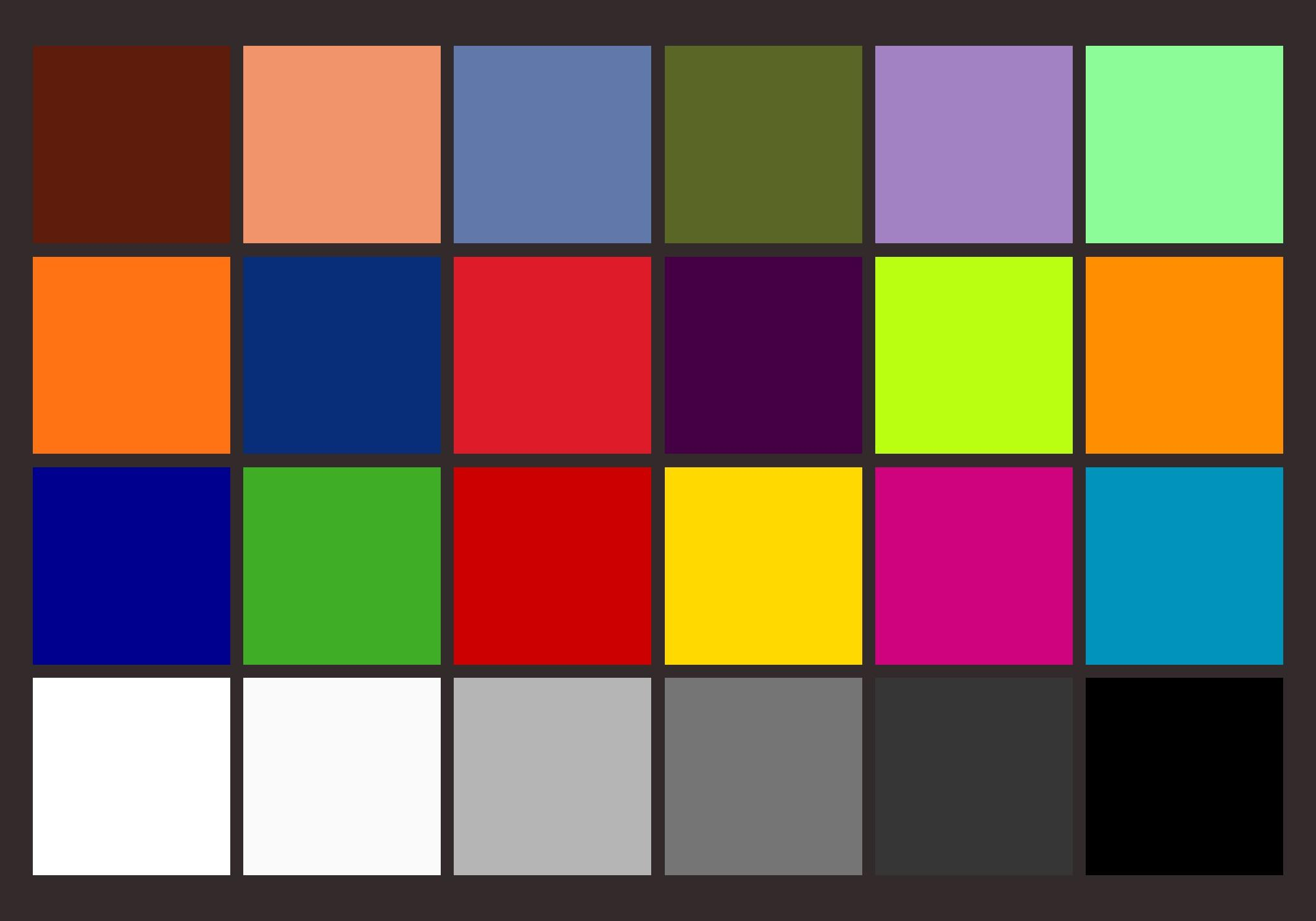## MTF 的测量方法

$$OTF(U,V)=\iint_{-\infty}^{+\infty}e^{-i\pi(xu+yv)}dxdy$$

$$OTF(u)=\int_{-\infty}^{+\infty}LSF(x)e^{-i2\pi ux}dx$$

$$ESF(x)=\int_{-\infty}^{x}LSF(x')dx'$$◎ PSF、LSF、ESF 和 MTF 之间的转换关系

## 色彩还原性测试原理

$$\Delta L^*=L_1^*-L_2^*$$

\begin{aligned} \Delta a^*&=a_1^*-a_2^*\\ \Delta b^*&=b_1^*-b_2^* \end{aligned}

$$\Delta E^*=(\Delta {L^*}^2+\Delta {a^*}^2+\Delta b^2)^{1/2}$$

$$\Delta C^*=(\Delta {a^*}^2+\Delta b^2)^{1/2}$$

$$\mathrm{White\ Error}=Max(R,G,B)-Min(R,G,B)$$

## 灰阶测试原理

$$Saturation=\frac{MaxGrey-MinGrey}{255}\times100$$

## 具体操作

### 下载 Imatest 软件

Imatest 是一款基于 MATLAB 的图像分析软件，功能十分强大。但巨坑的是这一款软件的试用版只能使用 20 次。这里的二十次不是说能够试用二十天，也不是打开关闭软件二十次，是分析图像二十次！如此巨坑的软件，在我安装后没有怎么用，并尝试了一下破解结果失败后，试用次数直接减到零次，差点吐血。后来为了完成此次实验，只好借用室友的电脑。

Imatest 的具体使用方法可以参考官方使用文档

### 拍摄图片

#### 拍摄 ISO12233 标准分别率板◎ IMG_0479.CR2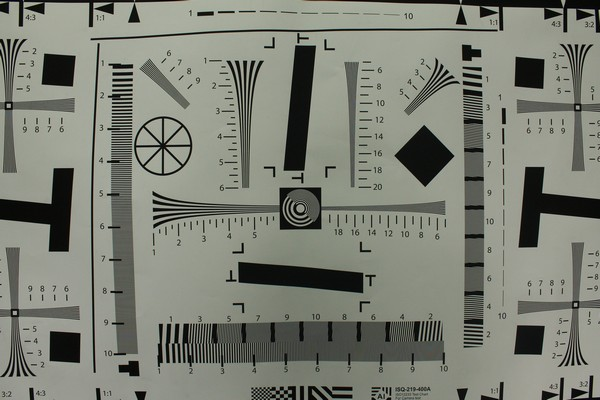◎ IMG_0482.CR2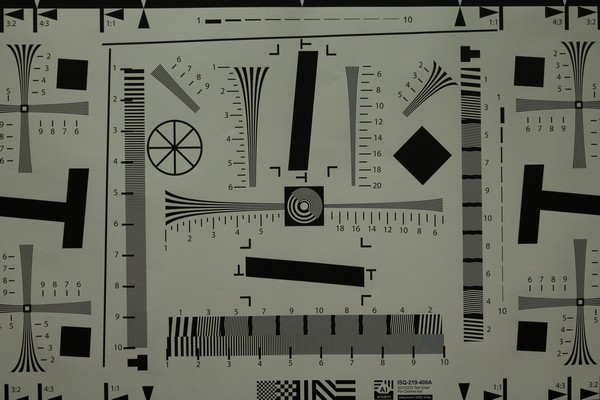◎ IMG_0483.CR2

#### 拍摄 X-rite 标准 24 色色卡◎ IMG_0487.CR2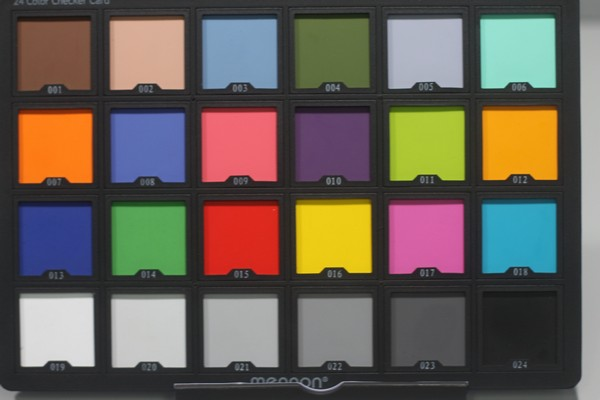◎ IMG_0488.CR2◎ IMG_0489.CR2

#### 拍摄灰阶测试卡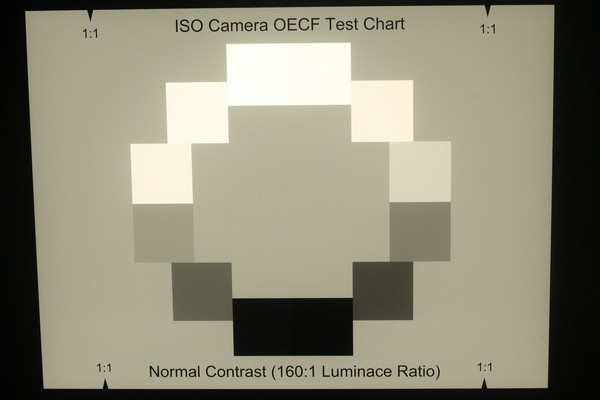◎ IMG_0492.CR2◎ IMG_0493.CR2

### 分析图像

#### MTF 曲线分析

##### 50mm 焦距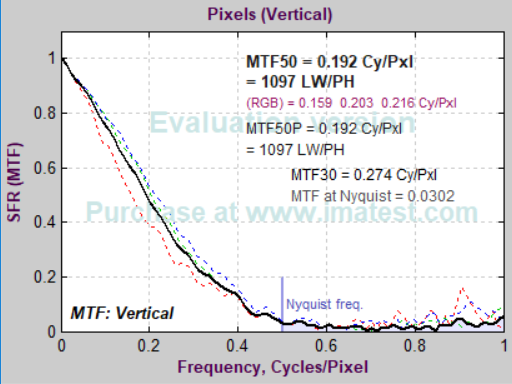◎ 视场中心◎ 视场边角

##### 18mm 焦距◎ 视场中心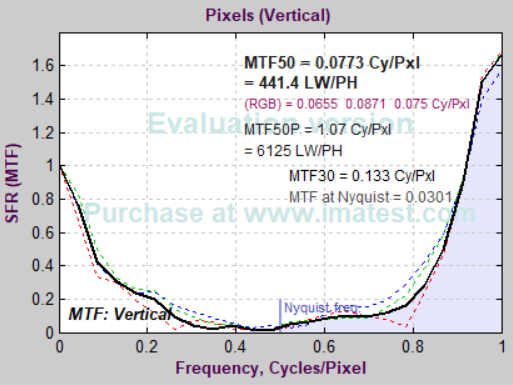◎ 视场边角

##### 75mm 焦距◎ 视场中心◎ 视场边角

MTF50 表示的是 MTF 为最大值的 50%（即 MTF=0.5）时，对应的空间频率。单位为 Cy/Pxl，则是表示在一个像素里有多少个线对，黑白线对为一个周期。由以上三组图线可知，IMG_0479 与 IMG_0482 的视场边角的 MTF 曲线在奈奎斯特频率到达 0.8 后骤增，分析其原因可能是由于在通过软件采样的时候，并没有采取到合适的位置，导致分析结果偏差很大,也可能是因为使用相对应的镜头拍摄时，没有拍摄好，成像质量较差。三张图的 MTF 曲线近似相等。在拍摄标准分辨率板的时候，房间光线不太充足，使用相机拍摄时没有将曝光时间调节到足够大，导致接受到的信号不足。

#### 色彩还原性与白平衡特性分析

##### A 光源◎ 色彩还原性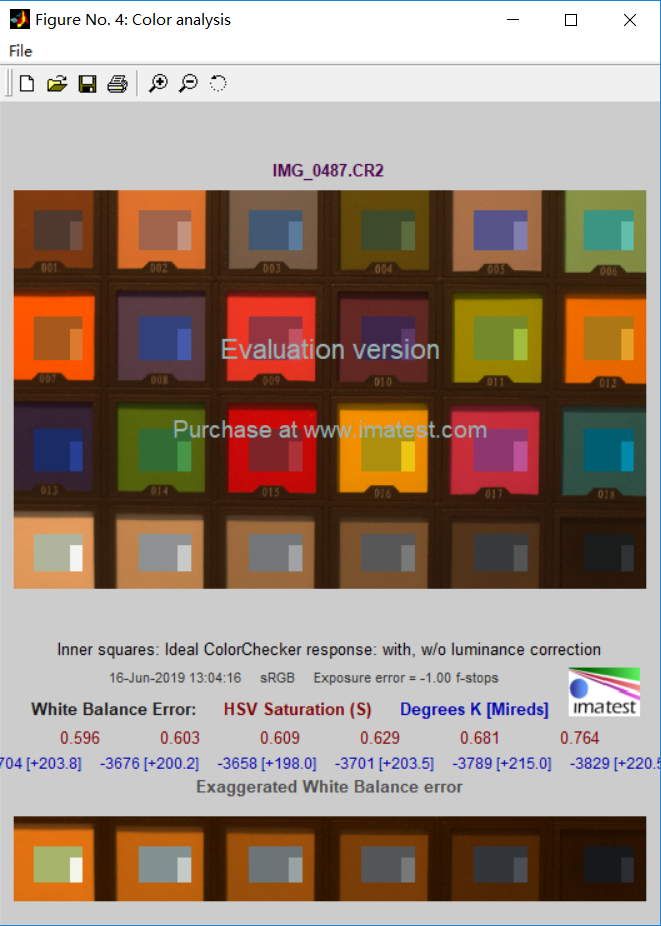◎ 白平衡特性

##### D65 光源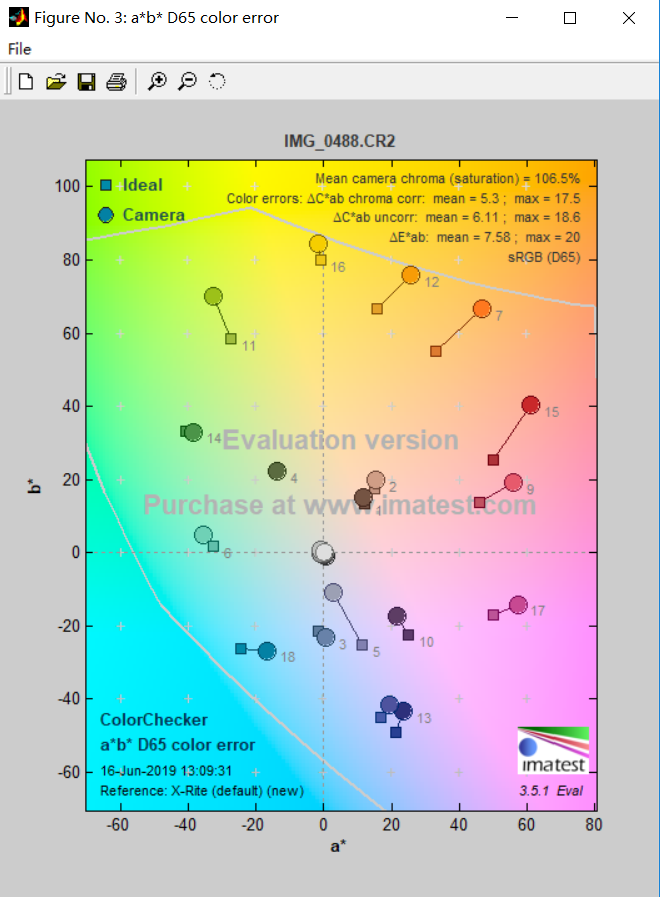◎ 色彩还原性◎ 白平衡特性

##### TL84 光源◎ 色彩还原性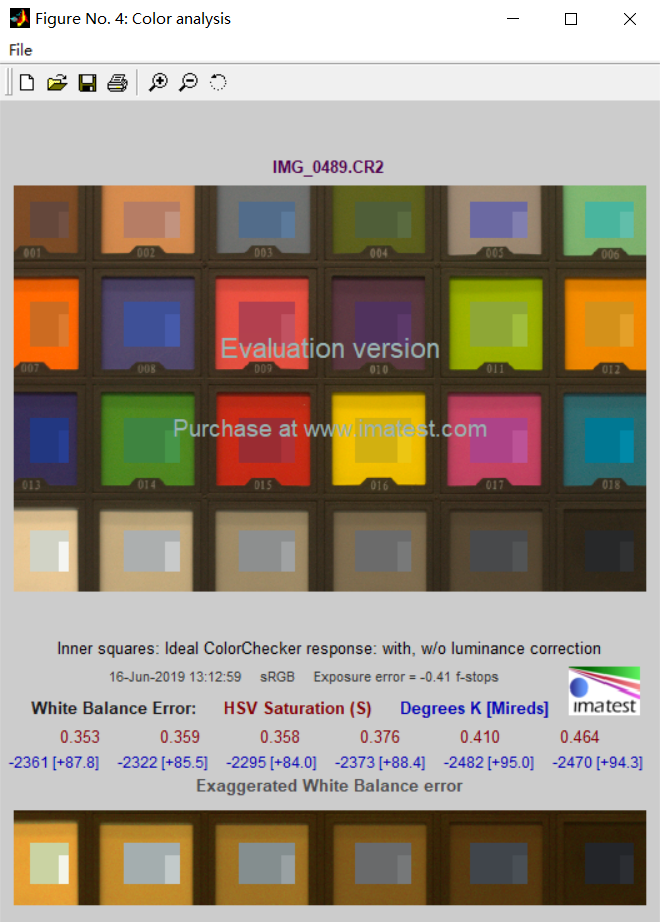◎ 白平衡特性

A 光源137.3%28.70.647
D65 光源106.5%5.30.014
TL84 光源120.4%19.30.387

#### 灰阶特性分析◎ IMG_0492◎ IMG_0493

IMG_0492 的动态范围较 IMG_0493 的动态范围大，所能表现得层次更为丰富，包含的色彩空间更广。

1. 对于这一领域相关概念的可以参考与「傅里叶光学」有关的教材和其他书籍。